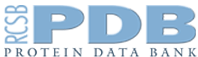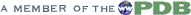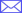An Information Portal to Biological Macromolecular Structures PDB Home | Contact UsSoftware Tools Home  |  Dictionary Home  |  PDBML Home  |  ISTABLE Home# ISTableUsage Example: V8-0-3

```----------------ISTable Usage Examples-------------

1. Creating an ISTable from scratch

2. Printing the table

3. Accessing rows

4. Accessing columns

5. Accessing cells

6. Searching the table

7. Deleting columns

8. Deleting rows

9. Replicating the table

#include "ISTable.h"

/*
** Example 1: How to create an ISTable from scratch.
** Note: This example uses dynamic allocation (heap)
*/

// Table name
string tableName;

// Create an empty table with case-sensitive column names.
ISTable* isTableP = new ISTable(tableName);

// Create three empty columns

vector<string> row;

// Prepare a row
row.push_back("one");
row.push_back("two");
row.push_back("three");

row.clear();

// Prepare a new row
row.push_back("four");
row.push_back("five");
row.push_back("six");

// Add the same row again

// Now the table is 3x3 (three rows and three columns)

/*
** Example 2: How to print an ISTable
*/

// Print the table
cout << (*isTableP) << endl;

/*
** Example 3: How to access table rows.
*/

// Get the third row (row index is 2) and print it out

isTableP->GetRow(row, 2);

cout << "The third row is: " << endl;
for (unsigned int rowI = 0; rowI < row.size(); ++rowI)
cout << row[rowI] << endl;

/*
** Example 4: How to access table columns.
*/

// Get the second column and print it out
vector<string> col;

isTableP->GetColumn(col, "secondColumn");

cout << "The second column is: " << endl;

for (unsigned int colI = 0; colI < col.size(); ++colI)
cout << col[colI] << endl;

/*
** Example 5: How to access table cells.
*/

// Get the cell in the first column and second row (row index is 1)
// and print it out
const string& cell = (*isTableP)(1, "firstColumn");

cout << "The cell in the first column and second row is: " << cell << endl;

/*
** Example 6: How to search the table.
*/

// Searching the table for rows that have values "four" and "six" in the
// first and the third column. Table cells are to be treated as
// case-sensitive strings

vector<string> searchCols;

// Set search column names first
searchCols.push_back("firstColumn");
searchCols.push_back("thirdColumn";

vector<string> searchValues;

// Initialize what is being searched for
searchValues.push_back("four");
searchValues.push_back("six");

// Indicate that the first search column content is to be treated as case
//   sensitive strings.
// Note that case sensitivity/insensitivity is determined only
//   from the first search column flags
isTableP->SetFlags("firstColumn", ISTable::DT_STRING | ISTable::CASE_SENSE);

// This vector will contain the rows that match the search criteria
vector<unsigned int> found;

// Search the table
isTableP->Search(found, searchValues, searchCols);

if (!found.empty())
{
// Found something. Print the rows that match.
cout << "Search found matching criteria in the following rows: " << endl;
for (unsigned int rowI = 0; rowI < found.size(); ++rowI)
cout << found[rowI] << endl;
}

// If only the first match is desired, use FindFirst()
unsigned int foundIndex = isTableP->FindFirst(searchValues, searchCols);
if (foundIndex == isTableP->GetNumRows())
{
// No match was found.
}
else
{
// First match found.
cout << "First match was found at row index: " << foundIndex << endl;
}

/*
** Example 7: How to delete columns.
*/

// Delete the second column
isTableP->DeleteColumn("secondColumn");

// Now the table is 3x2 (three rows and two columns)

/*
** Example 8: How to delete rows.
*/

// Delete the second row (row index is 1)
isTableP->DeleteRow(1);

// Note that the row index of the third row (row index 2) prior to
// deletion has now become 1

// Now the table is 2x2 (two rows and two columns)

/*
** Example 9: How to clone the table (copy to another one).
*/

// Copying the content of one table into the second one
// Both tables will have the same name, column names, number of rows and
// identical content.

ISTable* isTableCopyP = new ISTable();

(*isTableCopyP) = (*isTableP);

// Print both tables
cout << "Original table: " << endl << (*isTableP) << endl;
cout << "Cloned table: " << endl << (*isTableCopyP) << endl;

```© RCSB PDB